• ## 函数句柄

千次阅读 2020-06-01 17:33:11
函数句柄 定义：函数句柄是MATLAB数据类型，包含用于引用函数的信息。 好处：【1】可以把函数句柄作为参数传递给另外一个函数。 【2】提高重复操作的性能。 【3】可将函数句柄保存在数据结构或文件中供以后使用 函数...
函数句柄
定义：函数句柄是MATLAB数据类型，包含用于引用函数的信息。
好处：【1】可以把函数句柄作为参数传递给另外一个函数。
【2】提高重复操作的性能。
【3】可将函数句柄保存在数据结构或文件中供以后使用
函数句柄有两种不同的类型，都用函数句柄算子@来创建
一、命名的函数句柄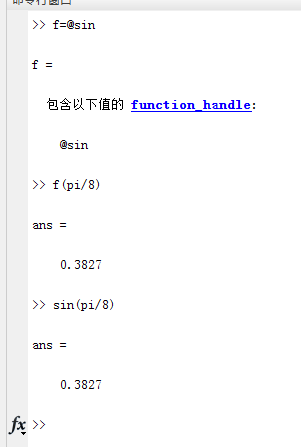二、使用匿名的函数句柄
通用格式为：
@（input-argument-list） expression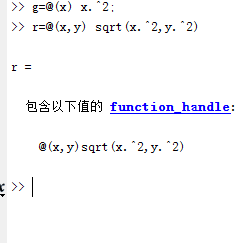g—计算输入值的平方
r----计算两个变量平方之和的平方根
可以像调用命名函数句柄那样调用匿名函数句柄。


展开全文• MATLAB 会将根据同一命名函数构造的函数句柄视为相同的函数句柄。isequal 函数在比较以下类型的句柄时会返回 true 值。 fun1 = @sin; fun2 = @sin; isequal(fun1,fun2) ans = logical 1 如果将这些句柄保存为 ...
比较根据命名函数构造的句柄
MATLAB 会将根据同一命名函数构造的函数句柄视为相同的函数句柄。isequal 函数在比较以下类型的句柄时会返回 true 值。
fun1 = @sin;
fun2 = @sin;
isequal(fun1,fun2)
ans =

logical

1

如果将这些句柄保存为 MAT 文件，以后将它们加载回工作区时它们仍然是相等的。
比较指向匿名函数的句柄
与指向命名函数的句柄不同，表示同一个匿名函数的函数句柄不相等。之所以将其视为不等，是因为 MATLAB 不能保证非参数变量的冻结值相同。例如，在本例中，A 是一个非参数变量。
A = 5;
h1 = @(x)A * x.^2;
h2 = @(x)A * x.^2;
isequal(h1,h2)
ans =

logical

0

如果您创建匿名函数句柄的副本，则副本与原始句柄相等。
h1 = @(x)A * x.^2;
h2 = h1;
isequal(h1,h2)
ans =

logical

1

比较嵌套函数的句柄
仅当您的代码在对包含嵌套函数的函数的同一调用中构造这些句柄时，MATLAB 才会将同一嵌套函数的函数句柄视为相等。此函数会构造两个指向同一嵌套函数的句柄。
function [h1,h2] = test_eq(a,b,c)
h1 = @findZ;
h2 = @findZ;

function z = findZ
z = a.^3 + b.^2 + c';
end
end

根据同一嵌套函数且在对其父函数的同一调用中构建的函数句柄被视为相等。
[h1,h2] = test_eq(4,19,-7);
isequal(h1,h2)
ans =

logical

1

使用不同调用构造的函数句柄被视为不相等。
[q1,q2] = test_eq(4,19,-7);
isequal(h1,q1)
ans =

logical

0



展开全文matlab
• ## MATLAB函数句柄

万次阅读 多人点赞 2019-05-15 10:44:51
感觉matlab函数句柄就是c语言里面的函数指针，在matlab里面叫它handle，句柄嘛，有了它就可以操纵这个对象(这里也可以叫做函数)，这个概念其实可以推广到很多东西，图形fig，自定义函数句柄(也就是下面将会展示的)，...

之前一直在用，也知道这么个东西，但是没怎么总结。感觉matlab函数句柄就是c语言里面的函数指针，在matlab里面叫它handle，句柄嘛，有了它就可以操纵这个对象(这里也可以叫做函数)，这个概念其实可以推广到很多东西，图形fig，自定义函数句柄(也就是下面将会展示的)，matlab自带函数句柄，以及某些函数返回的函数句柄，某些类对象或者表达式，也可以叫做句柄。

1、何为函数句柄？
函数句柄也是MATLAB中的一种常见的数据类型，
它的地位类似于其它计算机语言里的函数对象(Javascript,Python)，函数指针(C++)，或者函数引用(Perl)。
它的作用是将一个函数封装成一个变量，使其能够像其它变量一样在程序的不同部分传递。。引入函数句柄可使函数调用变得更加灵活方便，极大地提高了函数调用的速度和效率。MATLAB中函数句柄的使用使得函数也可以成为输入变量，并且能很方便地调用，提高函数的可用性和独立性。
2、函数句柄的创建：
方式①：直接加@
语法：@函数名fun1 = @sin;
方式②：str2func函数
语法：str2fun(‘函数名’)
fun2 = str2func(‘cos’);
方式③：str2func函数
语法：@(参数列表)单行表达式
fun3 = @(x, y)x.^2 + y.^2;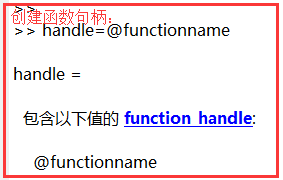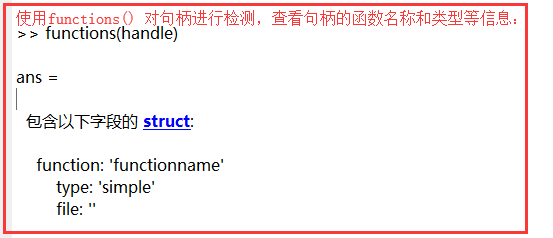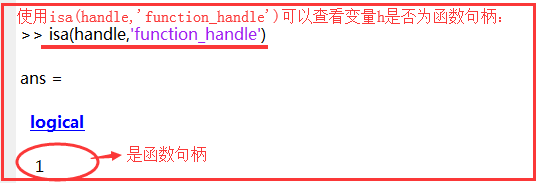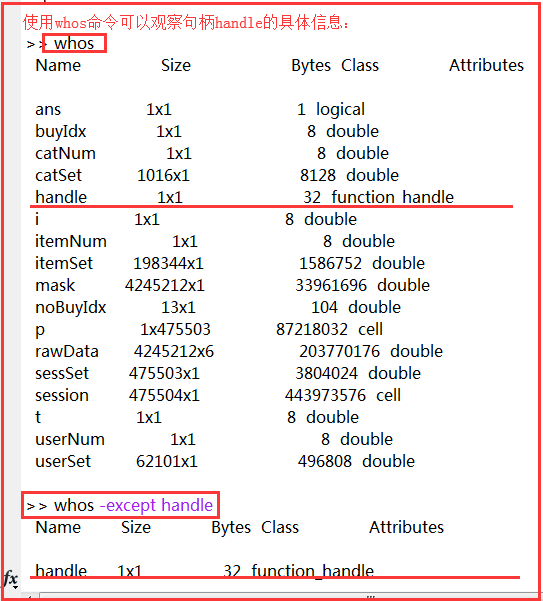3、函数句柄的调用：
假定一个函数的调用格式为：[y1,y2,…,yn] = FunctionName(x1,x2,…,xm)
该函数通过以下方式构建函数句柄：Hfunction = @FunctionName
则通过函数句柄实现函数运算的调用格式是：直接调用 Hfunction (x1,x2,…,xm) 或者 [y1,y2,…,yn]=feval (Hfunction,x1,x2,…,xm)
例如：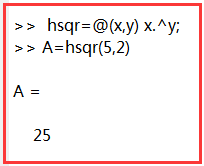句柄是matlab语言独有的参数，相当于C语言的指针。
句柄分为多种，如函数柄，对象柄，图形柄等。
图形句柄就指一个图形，在生成图形时同时得到一代号，如语句 h=plot(x,y),h 就是一个图形句柄，在后来的某一个地方就可用h代表这个图，如 set(h,…)，对这个图形进行再设置。
1.函数句柄
函数句柄简介：函数句柄(function handle)是MATLAB中的一类特殊的数据结构，它的地位类似于其它计算机语言里的函数对象(Javascript,Python)，函数指针(C++)，或者函数引用(Perl)。作用是将一个函数封装成一个变量，使其能够像其它变量一样在程序的不同部分传递。
句柄创建：
（1）fun1=@sin;
(2)fun2=str2fun(‘cos’);
(3)匿名函数：fun3=@(x,y)x2+y2;
函数句柄应用：函数句柄作为函数参数；利用函数句柄绘图；利用函数句柄滤波等
比如定义f(x)=x^2写
f=@(x)(x.^2)
其@(x)(x.^2)匿名函数第括号面自变量第二括号面表达式@函数指针
f=@(x)(x.2)表示匿名函数@(x)(x.2)赋值给f于f表示该函数
于f(2)=2.2=4；f(1:3)=[1:3].2=[1 4 9]等等
定义匿名函数调用别匿名函数比
f1=@(x,y)(x.2+y.2)
定义函数x2+y2
f2=@(t)(f1(t,2))
定义函数t^2+4
f3=@(x)(f1(x(1),x(2)))
定义函数x(1)2+x(2)2
使用匿名函数定要注意函数本身参数形式
f1(2,3)
表示22+32
f2(3)=3
表示3^2+4
f3([1,2])
表示12+22
说白函数指针
handle=@functionname
返指向制定MATLAB函数句柄
函数句柄指指向函数量句柄函数获函数句柄存
图形句柄和图形之间是一种一一对应关系，简单的说图形句柄就是指向了这个图形的一个变量，通过它可以设定该图形的各种属性。
matlab其实还有很多新颖奇妙的函数用法，给我们带来了很多便利，需要的时候多去查查就好了。
参考文献：
https://www.cnblogs.com/shenxiaolin/p/9257608.html
https://blog.csdn.net/algorithm_lixuan/article/details/75675176
https://jingyan.baidu.com/article/ed15cb1b58587c1be3698102.html
https://blog.csdn.net/daaikuaichuan/article/details/77801780
https://www.cnblogs.com/weiweicf/p/5970497.html
https://blog.csdn.net/daaikuaichuan/article/details/77801780
https://blog.csdn.net/yhl_leo/article/details/50699990
https://blog.csdn.net/lqzdreamer/article/details/70237495


展开全文• 函数句柄的创建和观察 函数句柄并不是伴随函数文件而自动形成的文件“属性”。它必须通过专门的定义才会生成。为一个函数定义句柄的方法有两种：或利用 @ 符号，或利用转换函数str2func。对函数句柄的内涵观察需...

函数句柄的创建和观察
函数句柄并不是伴随函数文件而自动形成的文件“属性”。它必须通过专门的定义才会生成。为一个函数定义句柄的方法有两种：或利用 @ 符号，或利用转换函数str2func。对函数句柄的内涵观察需借助专门指令functions 实现。
为MATLAB的“内建”函数创建函数句柄，并观察其内涵。
（1）创建
hsin=@sin
（2）类型判别
class(hsin)
size(hsin)
（3）借助指令functions观察内涵
CC=functions(hsin)
〖说明〗
l  指令hsin=@sin 的功能，可以用hsin=str2func('sin') 替代。
l  在创建一个函数句柄时，只有那函数正处在“视野Scope”内，此创建的函数句柄才是有效的。
所谓视野：在当前目录下，指令help, lookfor 搜索函数的范围。
l  定义函数句柄时，所指定的函数名不应包括“路径信息”，也不应包括扩展名。函数名最多只能包括63个字符。

函数句柄的基本用法
本节介绍：如何通过函数句柄执行相应函数的计算。假设，一个函数的调用格式为
[argout1, …, argoutn]=FunName(argin1, …, arginn)
又假设该函数的句柄Hfun已经存在，那么通过函数句柄实现函数运算的调用格式是：
[argout1, …, argoutn]=feval(Hfun,argin1, …, arginn)
〖说明〗
l  不管名为FunName的函数文件是否在当前搜索路径上，不管它是否在当前视野内，不管它是否子函数或私用函数，只要句柄Hfun已经被有效创建，那末它代表的函数总能被正确地执行。
l  在FunName存在重载函数时，借助句柄Hfun 的计算总能被“恰当地”执行。也就是说，它也会根据待计算的数据类型，从其包含的所有重载函数中选择相应的函数文件执行运算。
l  在不使用函数句柄的情况下，对 FunName 进行多次调用时，每次都要为该函数进行全面的路径搜索，这直接影响计算速度。借助Hfun可完全克服这种无谓的时间消耗。
【例6.6-2】本例通过sin函数及其句柄演示若干基本用法。
（1）函数句柄的创建
fhandle=str2func('sin');
（2）函数句柄在“数值计算”中的情况
ys=sin(pi/4)             %函数直接调用
yfold=feval('sin',pi/4)     %feval指令的“老式”调用
yfnew=feval(fhandle,pi/4) %feval指令的“新式”调用
查看是否存在文件：
which('function_name')

转载于:https://www.cnblogs.com/jocobHerbertPage/archive/2012/09/26/2704822.html
展开全文• matlab函数句柄 直接调用函数：  被调用函数只能被其M文件同名的主函数或在M文件中的其他函数调用，一个文件只有一个主函数。 间接调用函数：  避免只能使用直接调用函数的情况，个人理解就是为一个函数起了一个...matlab
• 本文演示如何创建指向局部函数句柄。如果函数返回指向局部函数句柄，则可以在主函数外部调用局部函数。此方法可让您在单个文件中包含多个可调用函数。 在您的工作文件夹下的 ellipseVals.m 文件中创建以下函数。...matlab
• 在MATLAB中，函数调用...对MATLAB库函数中提供的各种M文件中的函数和用户自己编写的程序中的内部函数都可以通过创建函数句柄实现这些函数的间接调用。创建函数句柄需要使用操作符@。 2、函数句柄的创建与调用 ...
•matlab
• 创建函数句柄的一般句法格式为： Function_Handle=@Function_Filename； 函数句柄的操作函数 functions(funhandle) 返回一个结构体，存储了函数的名称、函数类型（simple或overloaded），以及函数M文件...
• ## 函数句柄matlab

千次阅读 2016-06-02 12:12:33
函数句柄是一种特殊的数据类型，它提供了间接调用的函数的方法，类似于c语言中的指针，只不过这里是指向一个函数。  函数句柄包含了函数的路径、函数名、类型及可能存在的重载方法，必须通过专门的定义创建，创建...matlab
• MATLAB 的函数句柄： 1、何为函数句柄？ 　函数句柄也是MATLAB中的一种常见的数据类型， 　它的地位类似于其它计算机语言里的函数对象(Javascript,Python)，函数指针(C++)，或者函数引用(Perl)。 　它的作用是将...
• 函数句柄MATLAB的数据类型，包含用于引用函数的信息。使用函数句柄的主要优点是可以在调用中把函数句柄作为参数传递给另一个函数。 函数句柄主要有两种不同的类型。第一种函数句柄类型是命名的函数句柄，也称简单地...matlab
• ## matlab中函数句柄

千次阅读 2018-03-30 08:25:51
1.函数句柄函数句柄简介：函数句柄(function handle)是MATLAB中的一类特殊的数据结构，它的地位类似于其它计算机语言里的函数对象(Javascript,Python)，函数指针(C++)，或者函数引用(Perl)。作用是将一个函数封装成...
• ## MATLAB 函数句柄

千次阅读 2019-03-11 22:21:34
答：表示f为函数句柄，@是定义句柄的运算符。f=@(x)acos(x) 相当于建立了一个函数文件： % f.m functiony=f(x) y=acos(x); 若有下列语句：xsqual=@(x)1/2.*(x==-1/2)+1.*(x&gt;-1/28&amp;x&lt;1/2)+1.2...
• 这是一种间接访问函数的手段，用户可以很方便的...匿名函数创建函数句柄： sqr=@(x) x.^2; 调用 y=@sin; z=y(pi/2) 传递 x=ode23(@lotka,ALPHA,BETA); 句柄的操作 函数的参数必须写成函数句柄的格式：@sin ...matlab
• 2、函数句柄——提供一种间接调用函数的方法。创建函数句柄需要用到操作符@。 创建函数句柄的一般句法格式： Function_Handle = @Function_Filename; (Function_Filename是函数所对应的M文件的名称或...
• 函数句柄在MATLAB使用过程中经常涉及到， 个人小小的汇总一下，有不足和错误之处还望大家多多包涵和指点补充 函数句柄的定义具体参见官方文档...matlab
• ## Matlab中的函数句柄@

万次阅读 多人点赞 2016-02-19 20:57:38matlab 句柄函数 匿名函数
• 作用：   将函数传递给另一个函数（function functions）。  指定回调函数。...例子：建立一个函数句柄 生成函数句柄：f=@zeros 计算2*2的零矩阵，语法为：f(2) %相当于使用 zeros(2) &gt;&g...MATLAB
• ## 函数句柄的理解

万次阅读 2013-12-19 17:04:24
觉得自己很少用函数句柄，但是经常遇到，所以在这里总结一下。 函数句柄：是包含了函数的路径、函数名、类型以及可能存在的重载方法。 函数句柄必须通过专门的定义创建的，而一般的图像的句柄是自动建立的。 ...JQuery
• 1.函数句柄的创建 函数句柄(function handle)是MATLAB中的一类特殊的数据结构，它的地位类似于其它计算机语言里的函数对象(Javascript,Python)，函数指针(C++)，或者函数引用(Perl)。作用是将一个函数封装成一个...matlab
• ## matlab函数句柄介绍

千次阅读 2017-07-21 21:41:56
答：表示f为函数句柄，@是定义句柄的运算符。f=@(x)acos(x) 相当于建立了一个函数文件： % f.m function y=f(x) y=acos(x); 若有下列语句：xsqual=@(x)1/2.*(x==-1/2)+1.*(x>-1/28&x 则相当于建立了一个函数文件...matlab...